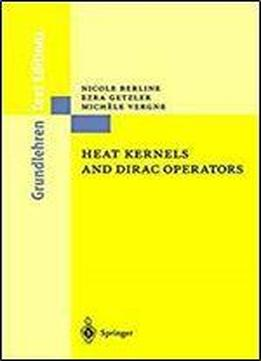# Heat Kernels And Dirac Operators (grundlehren Der Mathematischen Wissenschaften) by Nicole Berline / 2003 / English / PDF

The first edition of this book presented simple proofs of the Atiyah-Singer Index Theorem for Dirac operators on compact Riemannian manifolds and its generalizations (due to the authors and J.-M. Bismut), using an explicit geometric construction of the heat kernel of a generalized Dirac operator the new edition makes this popular book available to students and researchers in an attractive softcover. The first four chapters could be used as the text for a graduate course on the applications of linear elliptic operators in differential geometry and the only prerequisites are a familiarity with basic differential geometry. The next four chapters discuss the equivariant index theorem, and include a useful introduction to equivariant differential forms. The last two chapters give a proof, in the spirit of the book, of Bismut's Local Family Index Theorem for Dirac operators.

views: 417